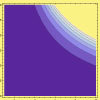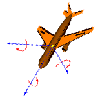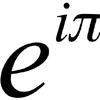# Resources tagged with: Complex numbers

Filter by: Content type:
Age range:
Challenge level:

There are 22 NRICH Mathematical resources connected to Complex numbers, you may find related items under The Number System and Place Value.

Broad Topics > The Number System and Place Value > Complex numbers### Complex Partial Fractions

##### Age 16 to 18Challenge Level

To break down an algebraic fraction into partial fractions in which all the denominators are linear and all the numerators are constants you sometimes need complex numbers.### Three by One

##### Age 16 to 18Challenge Level

There are many different methods to solve this geometrical problem - how many can you find?### 8 Methods for Three by One

##### Age 14 to 18Challenge Level

This problem in geometry has been solved in no less than EIGHT ways by a pair of students. How would you solve it? How many of their solutions can you follow? How are they the same or different?. . . .##### Age 14 to 18Challenge Level

This collection is designed to give an introductory taste of complex numbers.### Strolling Along

##### Age 14 to 18Challenge Level

What happens when we multiply a complex number by a real or an imaginary number?### Opening the Door

##### Age 14 to 18Challenge Level

What happens when we add together two complex numbers?### Sheep in Wolf's Clothing

##### Age 16 to 18Challenge Level

Can you work out what simple structures have been dressed up in these advanced mathematical representations?### Impedance Can Be Complex!

##### Age 16 to 18Challenge Level

Put your complex numbers and calculus to the test with this impedance calculation.### Pumping the Power

##### Age 16 to 18Challenge Level

What is an AC voltage? How much power does an AC power source supply?### What Are Numbers?

##### Age 7 to 18

Ranging from kindergarten mathematics to the fringe of research this informal article paints the big picture of number in a non technical way suitable for primary teachers and older students.### Two and Four Dimensional Numbers

##### Age 16 to 18Challenge Level

Investigate matrix models for complex numbers and quaternions.### Sextet

##### Age 16 to 18Challenge Level

Investigate x to the power n plus 1 over x to the power n when x plus 1 over x equals 1.### Footprints

##### Age 16 to 18Challenge Level

Make a footprint pattern using only reflections.### What Are Complex Numbers?

##### Age 16 to 18

This article introduces complex numbers, brings together into one bigger 'picture' some closely related elementary ideas like vectors and the exponential and trigonometric functions and. . . .### Complex Sine

##### Age 16 to 18Challenge Level

Solve the equation sin z = 2 for complex z. You only need the formula you are given for sin z in terms of the exponential function, and to solve a quadratic equation and use the logarithmic function.### Thousand Words

##### Age 16 to 18Challenge Level

Here the diagram says it all. Can you find the diagram?### Cube Roots

##### Age 16 to 18Challenge Level

Evaluate without a calculator: (5 sqrt2 + 7)^{1/3} - (5 sqrt2 - 7)^1/3}.### Napoleon's Theorem

##### Age 14 to 18Challenge Level

Triangle ABC has equilateral triangles drawn on its edges. Points P, Q and R are the centres of the equilateral triangles. What can you prove about the triangle PQR?### Complex Rotations

##### Age 16 to 18Challenge Level

Choose some complex numbers and mark them by points on a graph. Multiply your numbers by i once, twice, three times, four times, ..., n times? What happens?### An Introduction to Complex Numbers

##### Age 16 to 18

A short introduction to complex numbers written primarily for students aged 14 to 19.### Target Six

##### Age 16 to 18Challenge Level

Show that x = 1 is a solution of the equation x^(3/2) - 8x^(-3/2) = 7 and find all other solutions.### Roots and Coefficients

##### Age 16 to 18Challenge Level

If xyz = 1 and x+y+z =1/x + 1/y + 1/z show that at least one of these numbers must be 1. Now for the complexity! When are the other numbers real and when are they complex?